# Year 4 Maths Printable Worksheets

Help your child build on what they've already learned with our year 4 maths worksheets. Covering topics like times tables, properties of 2D and 3D shapes, estimating, measuring and recording results, fractions, decimals, mental maths and more, your child will find a variety of resources in our year 4 maths worksheets.

Filters

### Classifying angles

Students are asked to write 'straight', 'acute', 'obtuse' or right below each angle. There is an example to help them.

Age: 8 - 9
1
2
3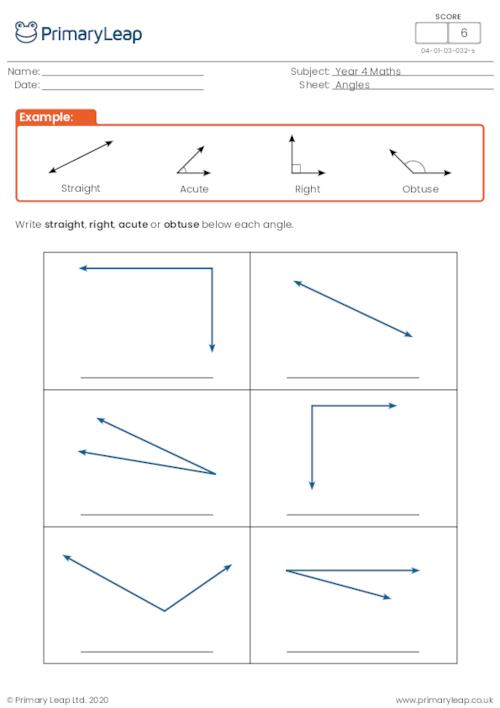### Adding 4-digit numbers in columns (with carrying)

This maths worksheet includes addition sums with two 4-digit numbers in columns. Students will practise carrying numbers.

Age: 8 - 9
1
2
3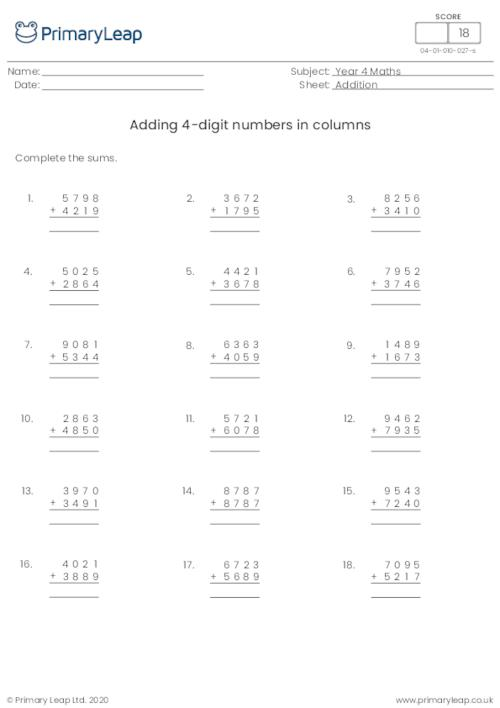### Subtraction with Roman Numerals to 50

Children practise their subtraction with these Roman numerals sums up to 50. There are 20 questions for students to answer, their answer should be given in Roman numerals.

Age: 8 - 9
1
2
3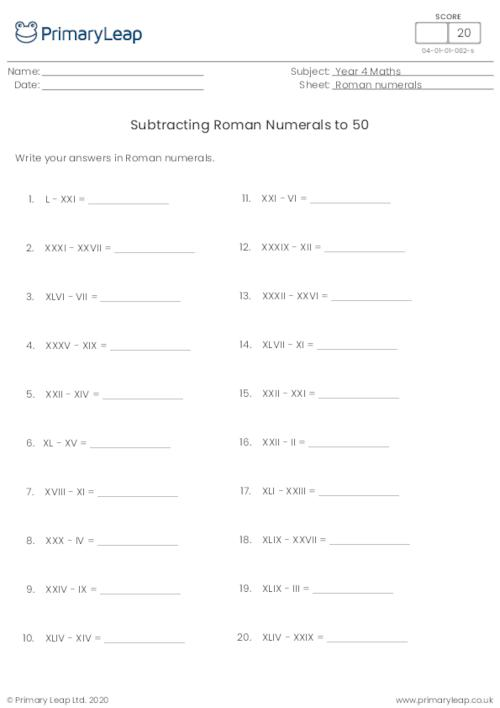### Addition with Roman Numerals to 50

Children practise their addition with these Roman numerals sums up to 50. There are 20 questions for students to answer, their answer should be given in Roman numerals.

Age: 8 - 9
1
2
3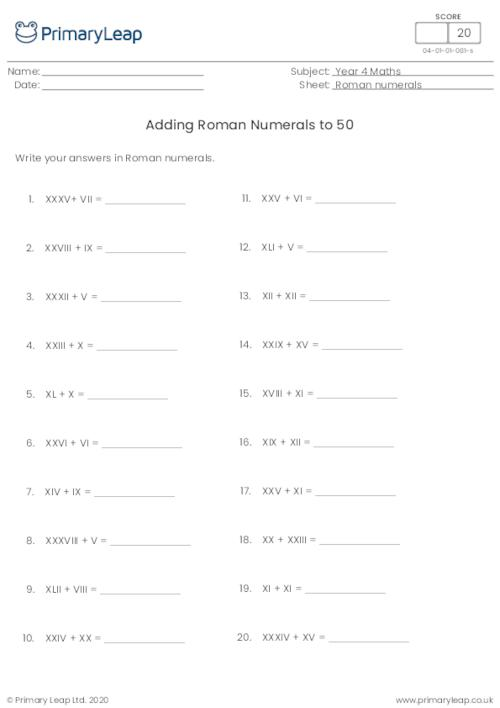### Subtraction with Roman Numerals to 20

Children practise their subtraction with these Roman numerals sums up to 20. There are 20 questions for students to answer, their answer should be given in Roman numerals.

Age: 8 - 9
1
2
3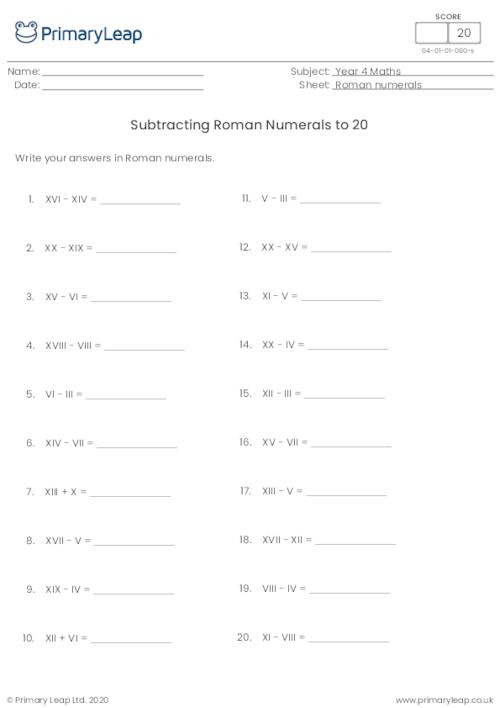### Addition with Roman Numerals to 20

Children practise their addition with these Roman numerals sums up to 20. There are 20 questions for students to answer, their answer should be given in Roman numerals.

Age: 8 - 9
1
2
3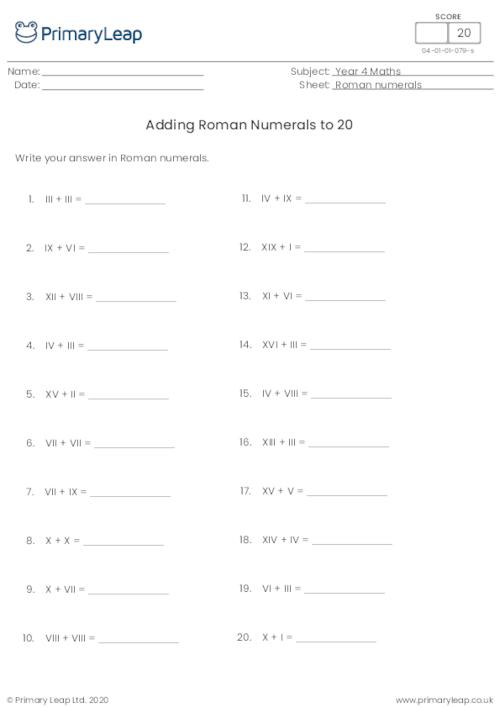### Writing Roman Numerals 50 - 100

Children write the numbers up to 100 as Roman numerals.

Age: 8 - 9
1
2
3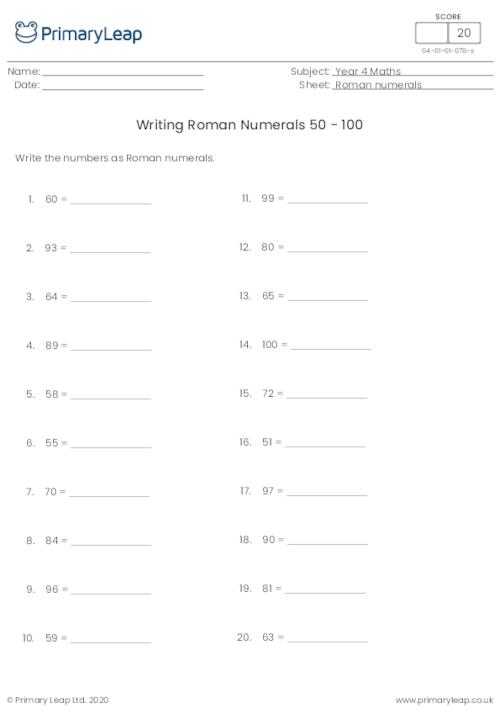### Reading Roman Numerals 50 - 100

Children read the Roman numerals up to 100 and write the number in normal form.

Age: 8 - 9
1
2
3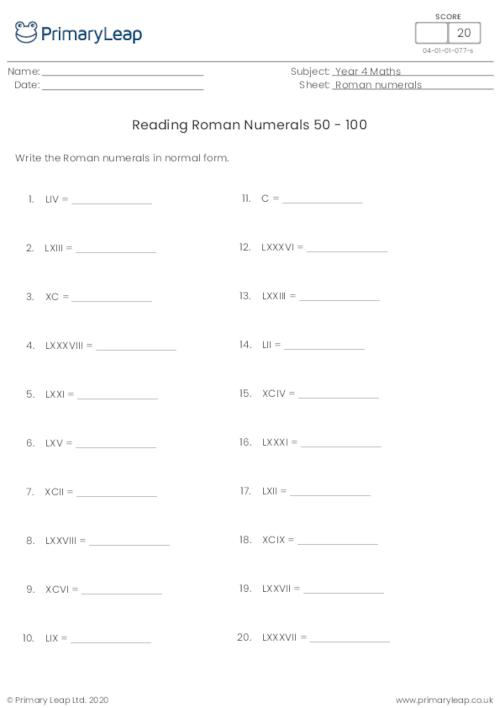### Writing Roman Numerals 1 - 50

Children write the numbers up to 50 as Roman numerals.

Age: 8 - 9
1
2
3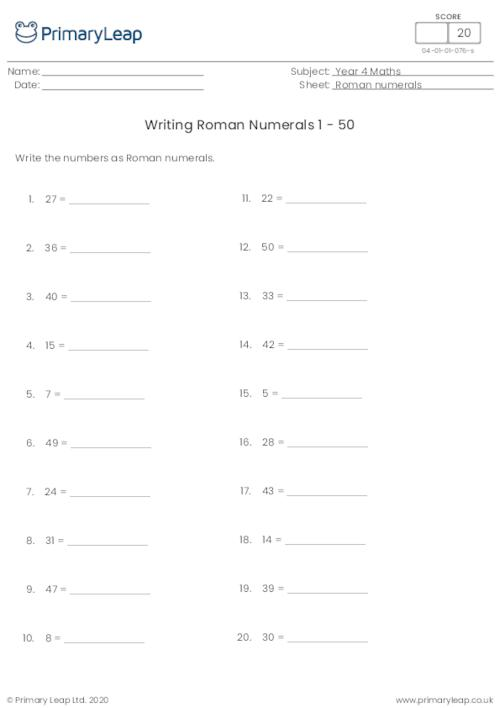### Writing Roman Numerals 1 - 20

Children write the numbers up to 20 as Roman numerals.

Age: 8 - 9
1
2
3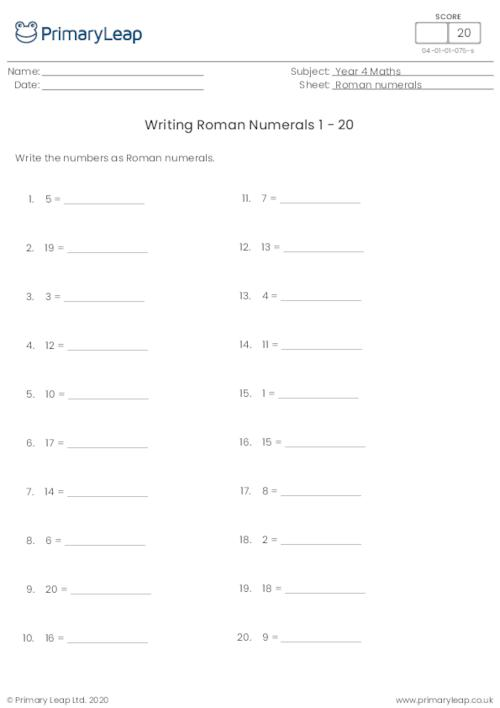### Reading Roman Numerals 1 - 50

Children read the Roman numerals up to 50 and write the number in normal form.

Age: 8 - 9
1
2
3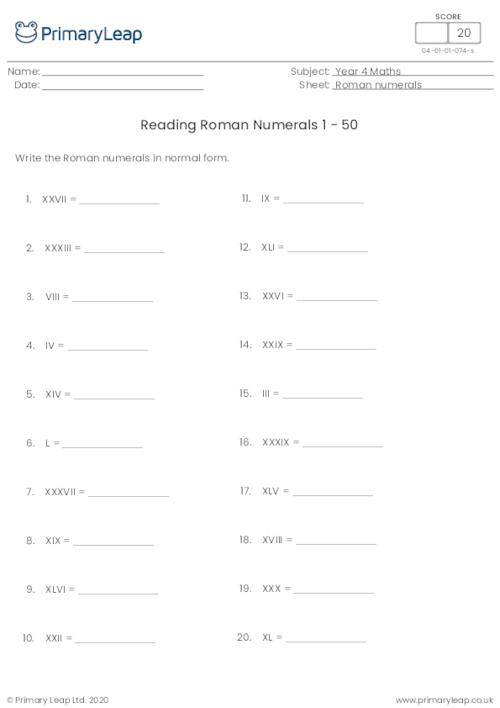### Reading Roman Numerals 1 - 20

Children read the Roman numerals and write the number in normal form.

Age: 8 - 9
1
2
3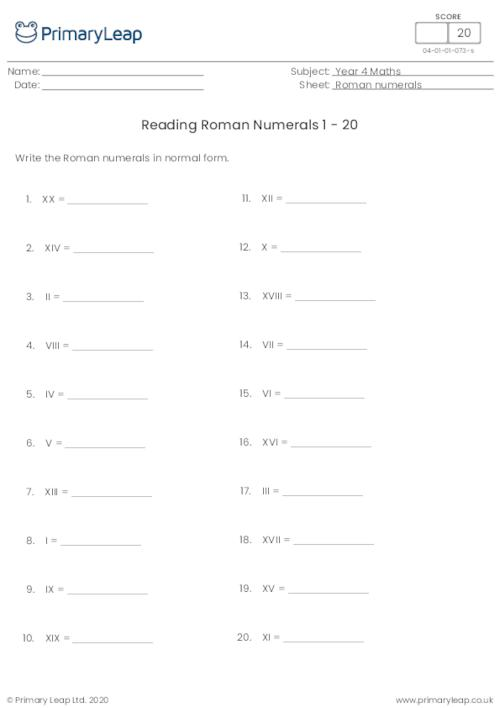### Roman Numerals Matching to 20

Children match the Roman numerals to the correct number. This maths resource helps children recognise Roman numerals up to 20. This is good practise before they start addition and subtraction sums with Roman numerals.

Age: 8 - 9
1
2
3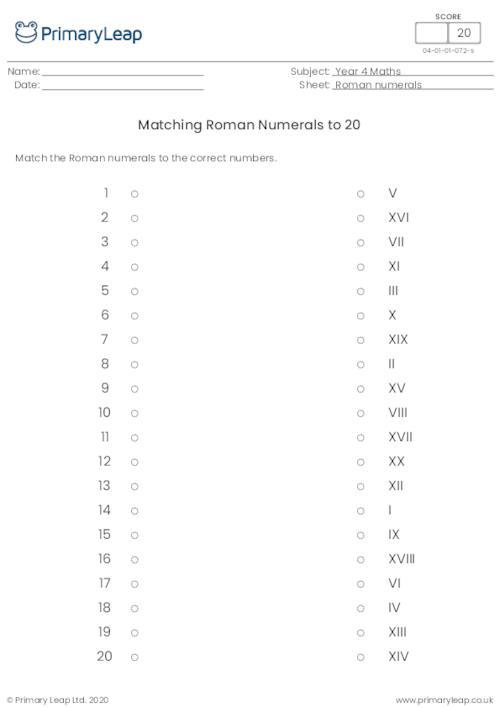### Roman Numerals Chart 50 - 100

This Roman numerals chart showing numbers 50 - 100 can be used as an aid to help children complete maths activities on Roman numerals.

Age: 8 - 9
1
2
3### Roman Numerals Chart 1 - 50

This Roman numerals chart showing numbers 1 - 50 can be used as an aid to help children complete maths activities on Roman numerals.

Age: 8 - 9
1
2
3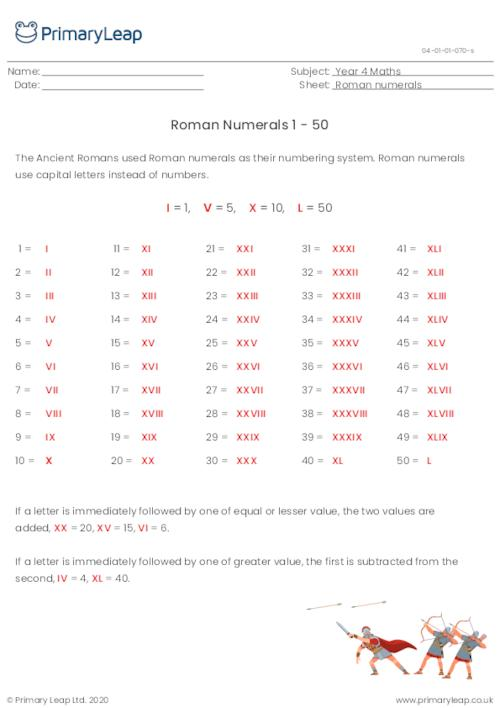### Roman Numerals Chart 1 - 20

This Roman numerals chart can be used as an aid to help children complete maths activities on Roman numerals.

Age: 8 - 9
1
2
3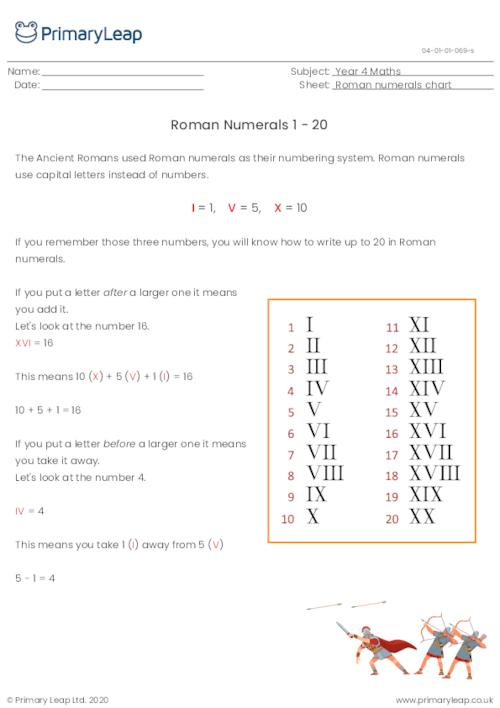### Types of Numbers

A KS2 maths resource on odd numbers, even numbers, prime numbers, square numbers and multiples.

Age: 8 - 9
1
2
3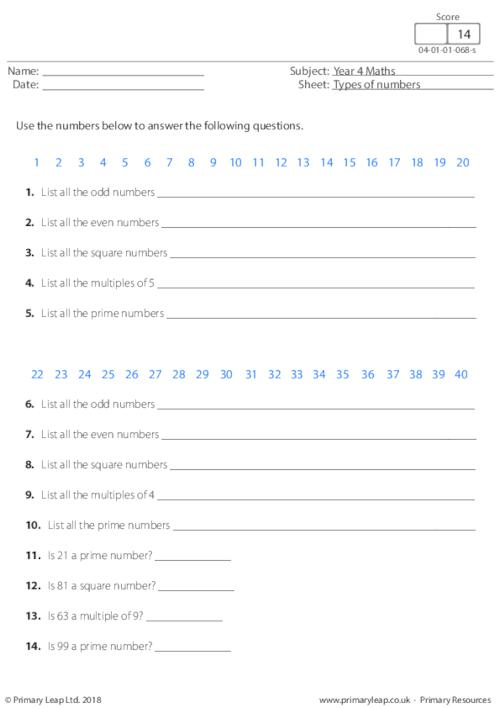### Equivalent Fractions

A KS2 maths resource on equivalent fractions.

Age: 8 - 9
1
2
3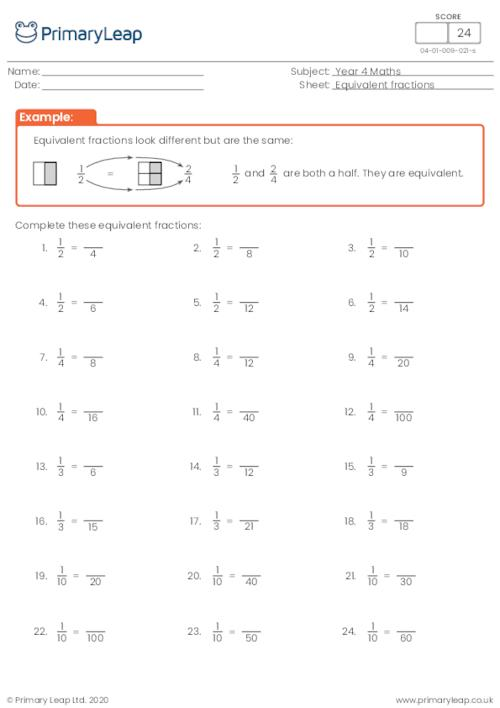### Bar Modelling - 12 xtable

Bar models can be used to solve different kinds of multiplication. Students answer these 12 xtable questions as quickly as possible.

Age: 8 - 9
1
2
3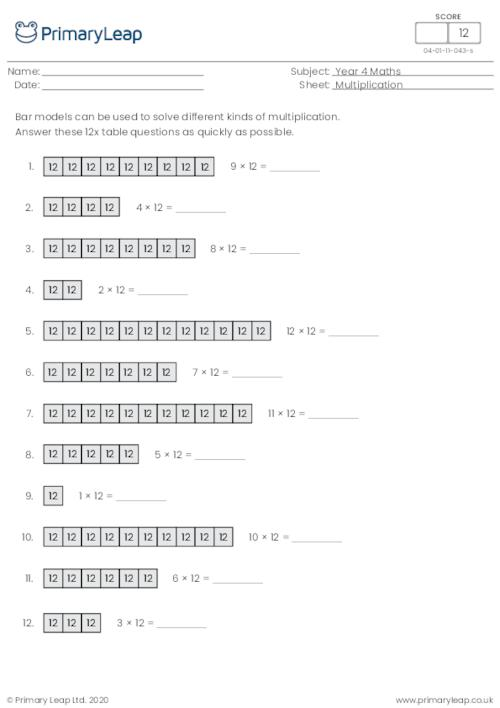### Bar Modelling - 11 xtable

Bar models can be used to solve different kinds of multiplication. Students answer these 11 xtable questions as quickly as possible.

Age: 8 - 9
1
2
3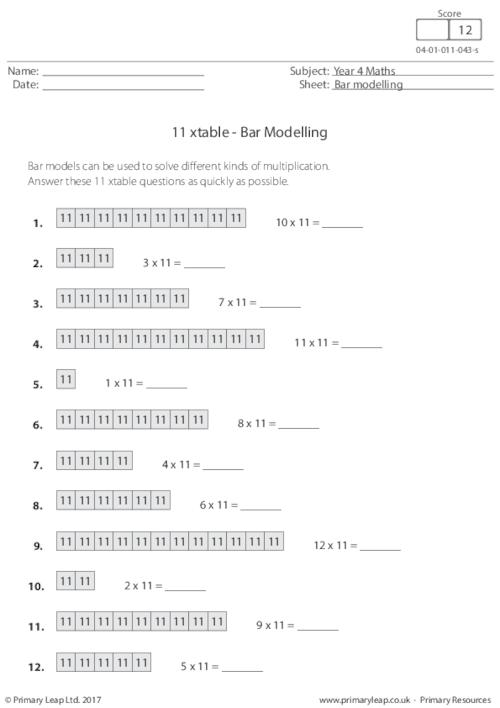### Bar Modelling - 6 xtable

Bar models can be used to solve different kinds of multiplication. Students answer these 6 xtable questions as quickly as possible.

Age: 8 - 9
1
2
3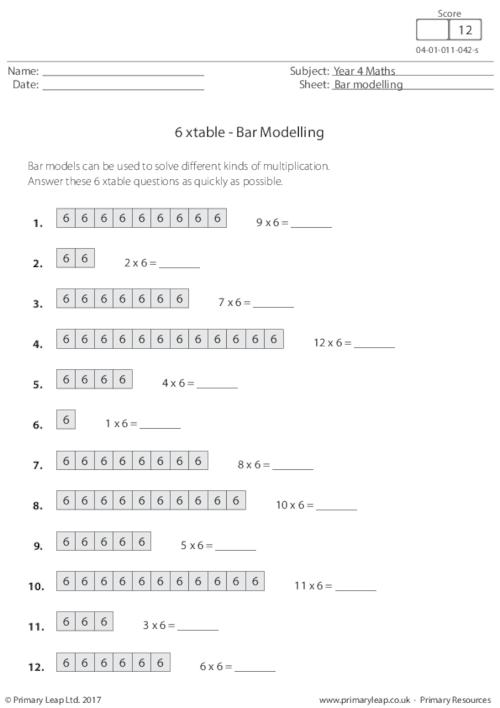### Bar Modelling - 7 xtable

Bar models can be used to solve different kinds of multiplication. Students answer these 7 xtable questions as quickly as possible.

Age: 8 - 9
1
2
3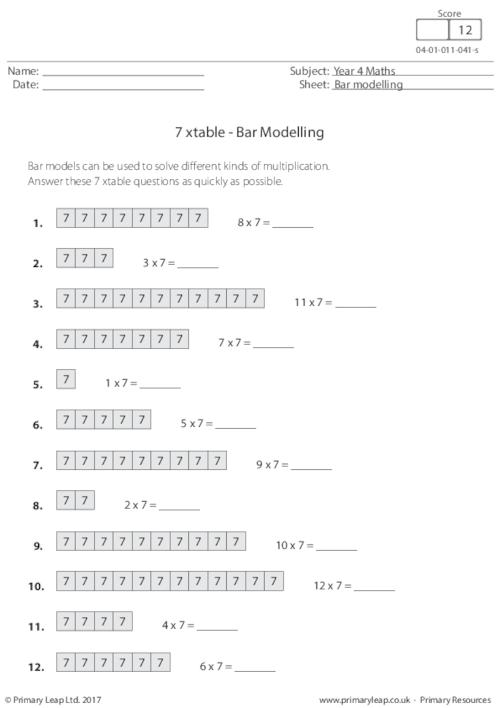### 1-Minute Maths - Multiplication (1)

This maths worksheet asks students to complete as many of the sums as they can in 1 minute. This will help a child's basic calculation skills and make more difficult maths easier for them later on.

Age: 8 - 9
1
2
3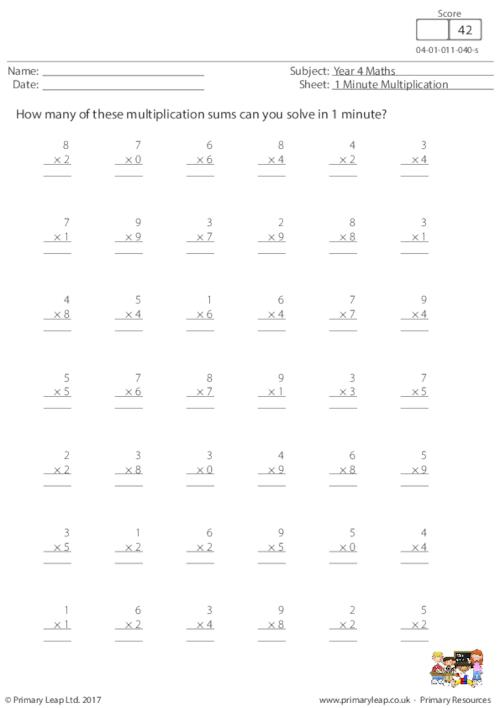### Lattice Multiplication: 2 by 2 digits (3)

Lattice multiplication is a method of multiplying numbers using a grid. This method breaks the multiplication process into smaller steps, which some students may find easier. There are 9 sums for students to work out.

Age: 8 - 9
1
2
3### Lattice Multiplication: 2 by 2 digits (2)

Lattice multiplication is a method of multiplying numbers using a grid. This method breaks the multiplication process into smaller steps, which some students may find easier.

Age: 8 - 9
1
2
3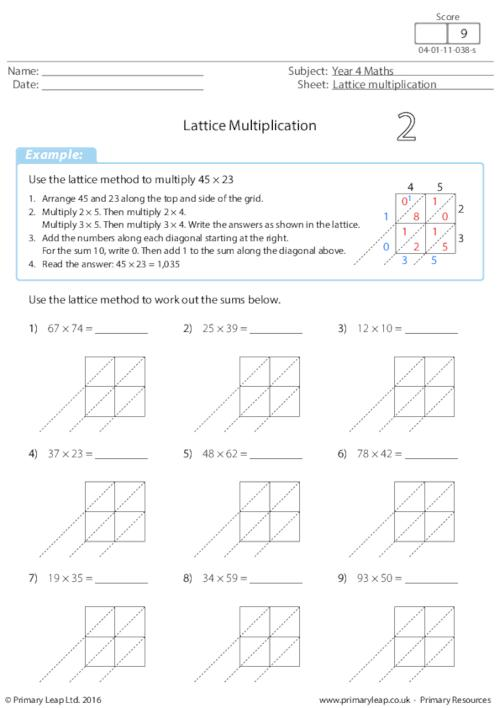### Lattice Multiplication: 2 by 2 digits (1)

Lattice multiplication is a method of multiplying numbers using a grid. This method breaks the multiplication process into smaller steps, which some students may find easier.

Age: 8 - 9
1
2
3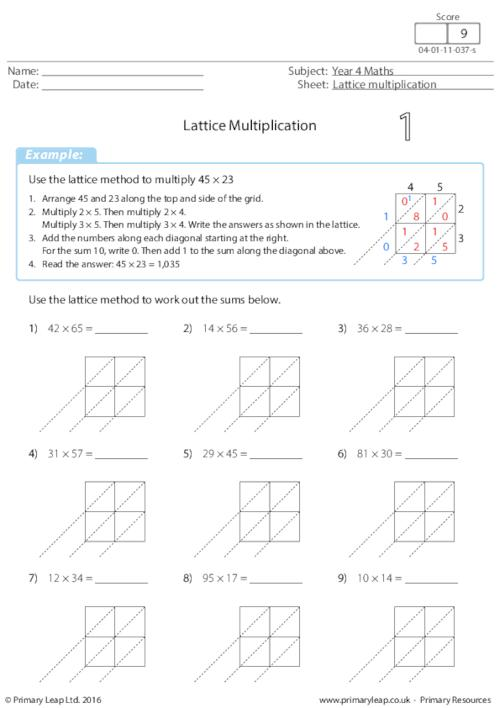### Identifying shapes - Octagons

Students are asked to colour a path to help Mr. Octopus find his way home. He can only follow a path of octagons.

Age: 8 - 9
1
2
3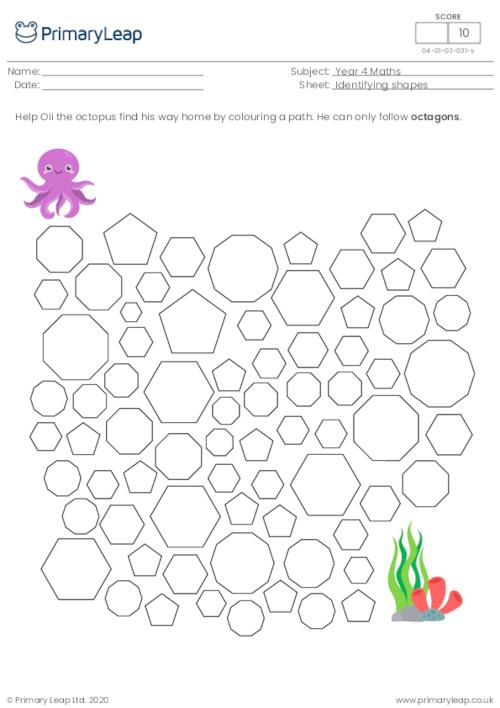### Maths word problems

Age: 8 - 9
1
2
3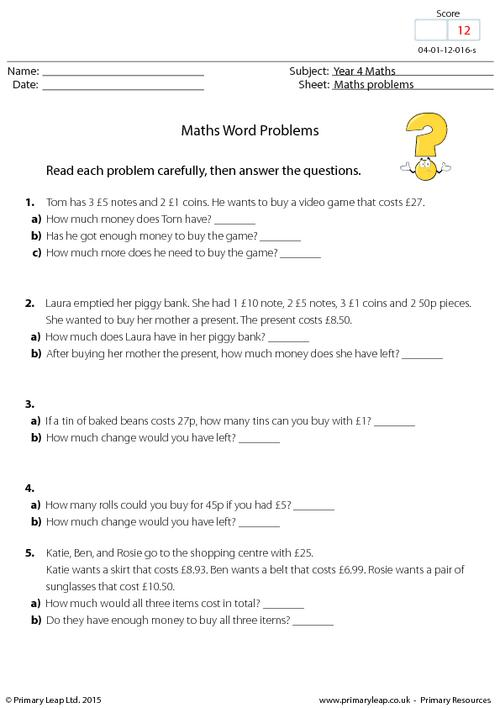### Solving the Equation - Multiplication and division

Maths: Students are asked to work out the value of each letter to complete the sum. There are 14 sums to work out.

Age: 8 - 9
1
2
3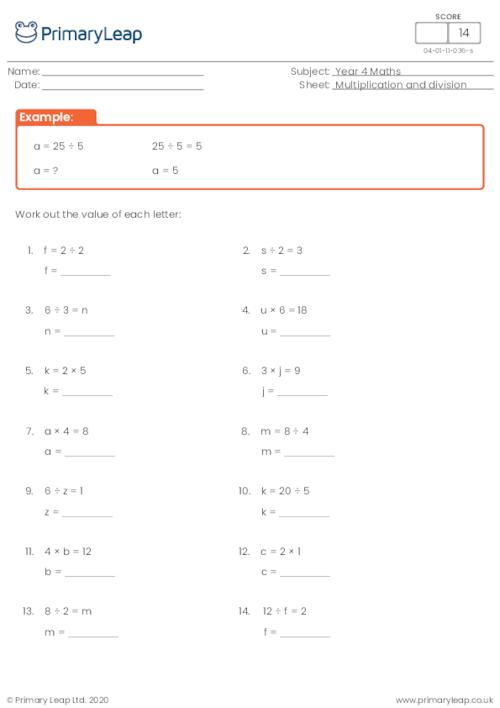### Solving the Equation - Addition and subtraction

Students are asked to work out the value of each letter to complete the sum. There are 14 sums to work out.

Age: 8 - 9
1
2
3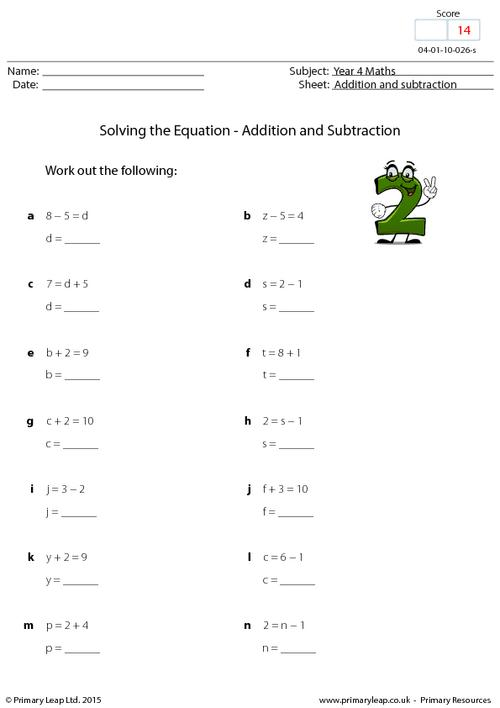### 25 and counting

Year 4 maths printable - This worksheet includes number sequences. Students are asked to fill in the missing numbers. The numbers are going up in 25s.

Age: 8 - 9
1
2
3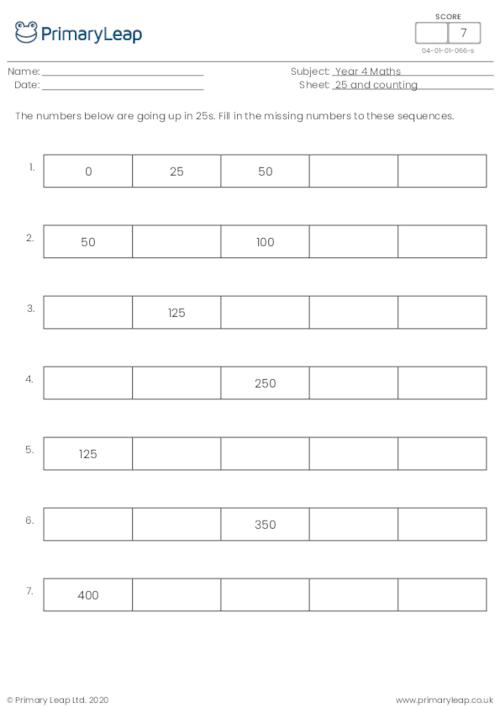### Sequences - Multiples of 6

A multiple of a number is a number which can be divided exactly by that number. 72 can be divided exactly by 6, so 72 is a multiple of 6. Students must complete the multiples of 5 sequences.

Age: 8 - 9
1
2
3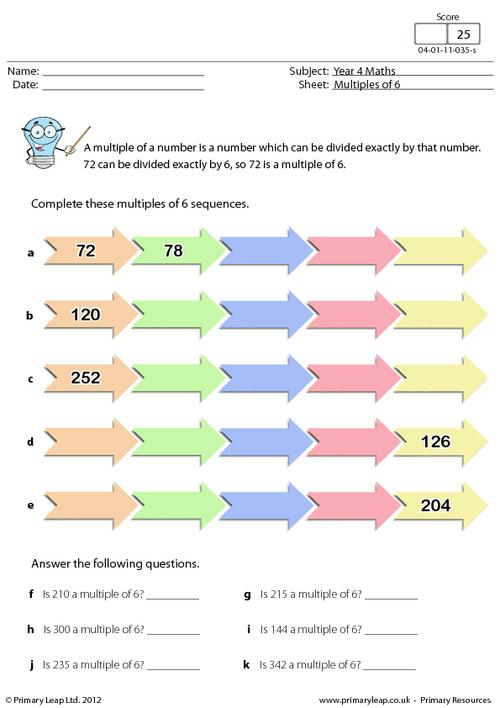### Sequences - Multiples of 5

A multiple of a number is a number which can be divided exactly by that number. 225 can be divided exactly by 5, so 225 is a multiple of 5. Students must complete the multiples of 5 sequences.

Age: 8 - 9
1
2
3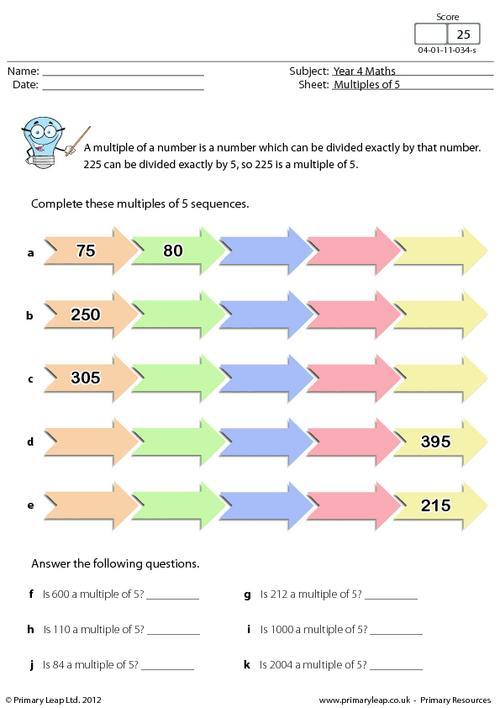### Sequences - Multiples of 4

A multiple of a number is a number which can be divided exactly by that number. 44 can be divided exactly by 4, so 44 is a multiple of 4. Students must complete the multiples of 4 sequences.

Age: 8 - 9
1
2
3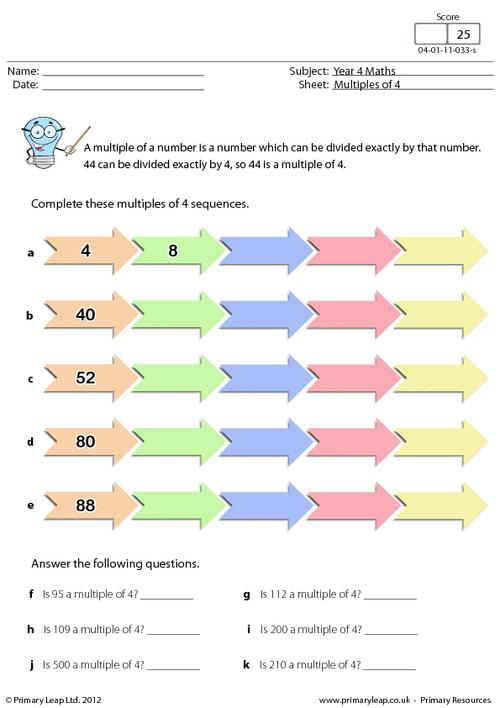### Decimal fractions

Year 4 Maths - This worksheet is all about decimal fractions. Students must first write the decimal fractions in words and then write the words in numbers.

Age: 8 - 9
1
2
3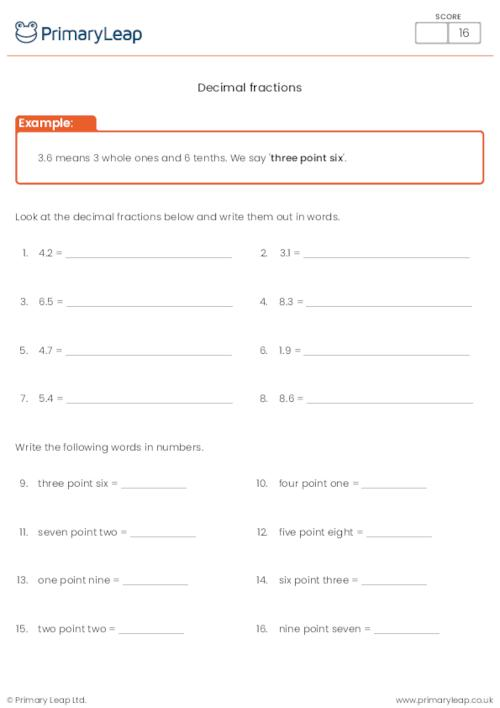### Times table practice

Year 4 Maths - Multiplication. This times table resource is great for learning your times tables. Set yourself a time limit and see how well you do!

Age: 8 - 9
1
2
3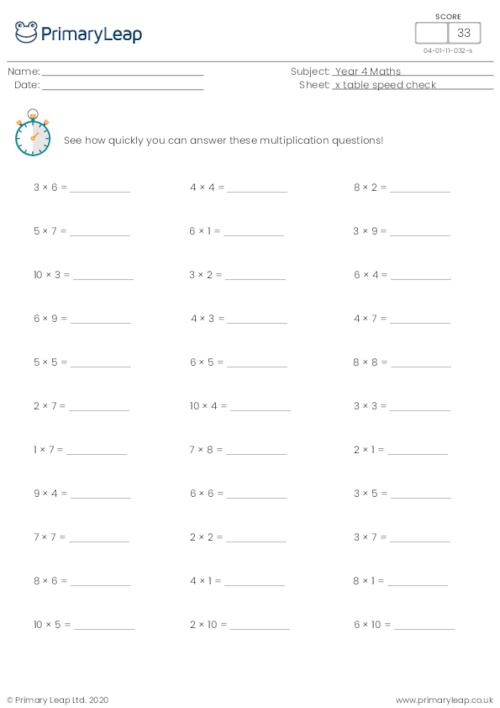### Division

Math: Division - Answer the two sets of division questions.

Age: 8 - 9
1
2
3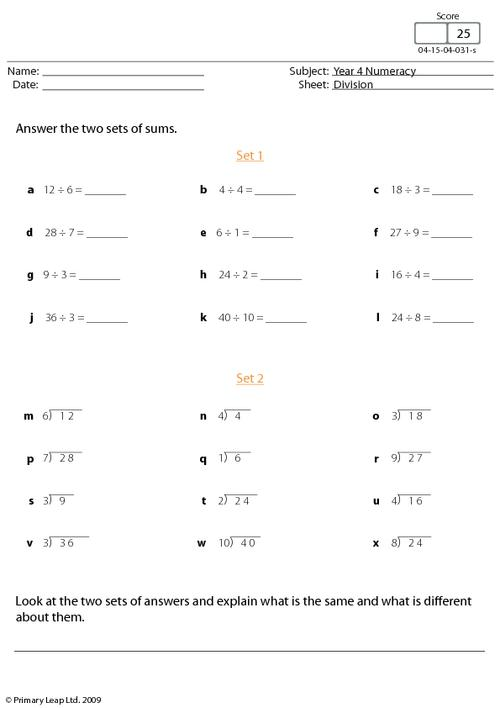### Subtraction

This worksheet includes 6 vertical subtraction questions and 4 subtraction questions with words.

Age: 8 - 9
1
2
3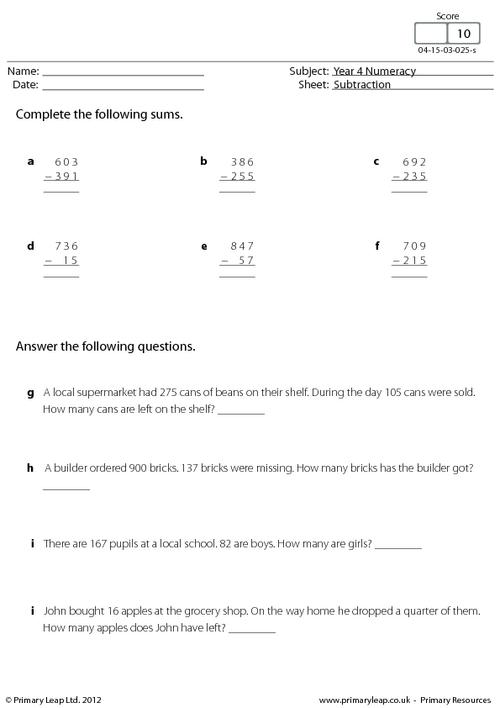### Fractions of shapes

Math: Fractions - Look at the shapes and write the fraction of circles in each group.

Age: 8 - 9
1
2
3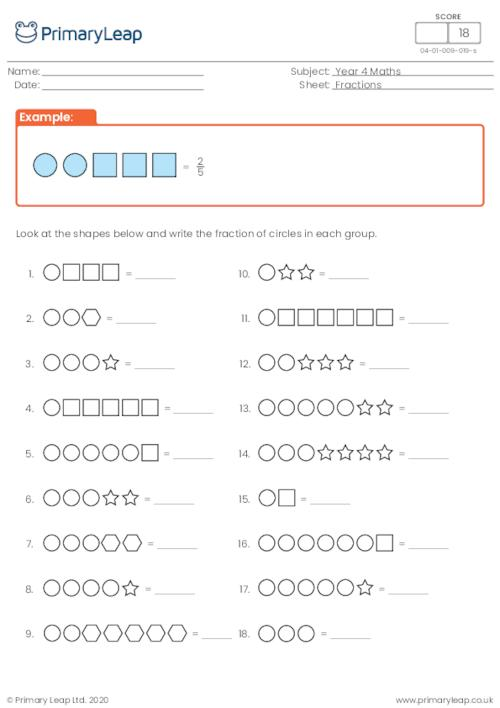### Mental arithmetic - Test 8

A year 4 numeracy worksheet with 12 mental arithmetic questions.

Age: 8 - 9
1
2
3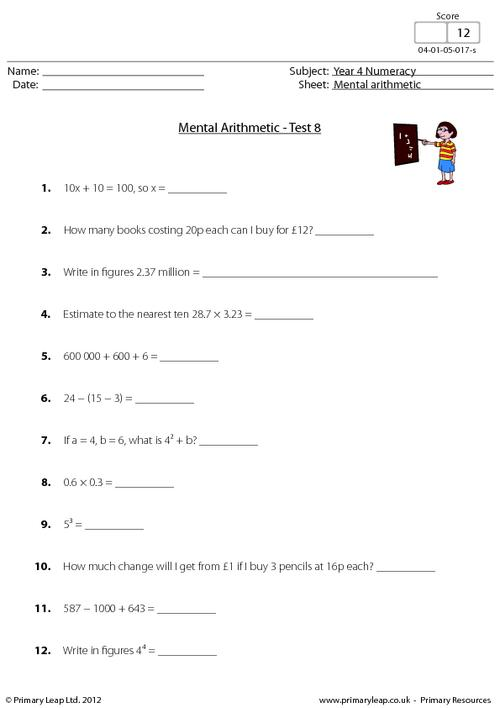### Mental arithmetic - Test 7

A year 4 numeracy worksheet with 12 mental arithmetic questions.

Age: 8 - 9
1
2
3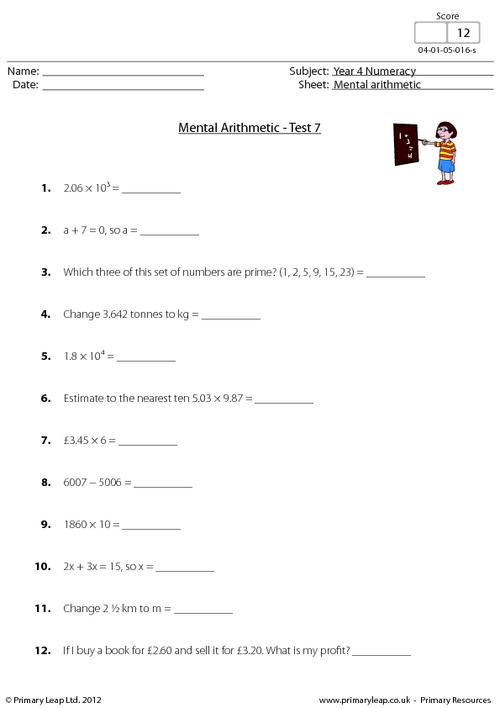### Mental arithmetic - Test 6

A year 4 numeracy worksheet with 12 mental arithmetic questions.

Age: 8 - 9
1
2
3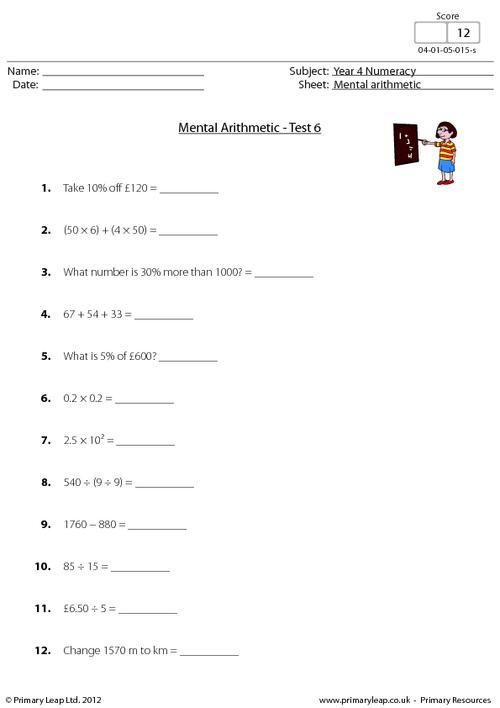### Mental arithmetic - Test 5

A year 4 numeracy worksheet with 12 mental arithmetic questions.

Age: 8 - 9
1
2
3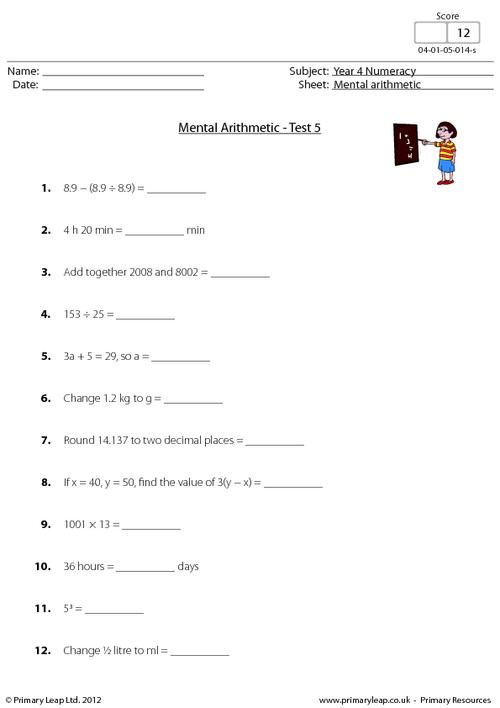### Mental arithmetic - Test 4

A year 4 numeracy worksheet with 12 mental arithmetic questions.

Age: 8 - 9
1
2
3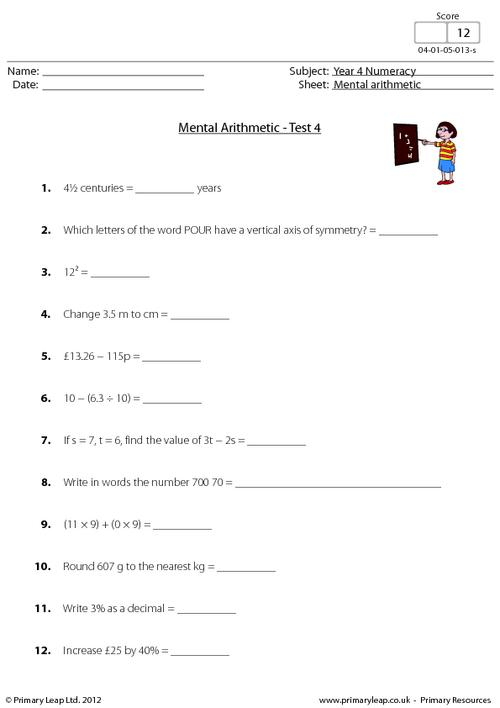### Mental arithmetic - Test 3

A year 4 numeracy worksheet with 12 mental arithmetic questions.

Age: 8 - 9
1
2
3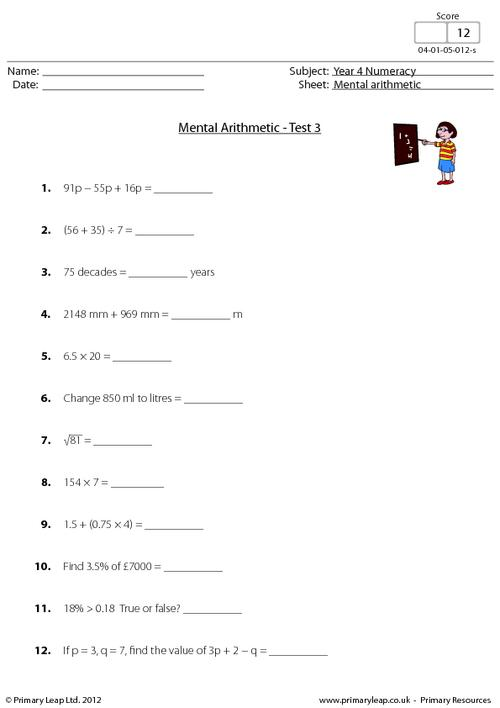### Mental arithmetic - Test 2

A year 4 numeracy worksheet with 12 mental arithmetic questions.

Age: 8 - 9
1
2
3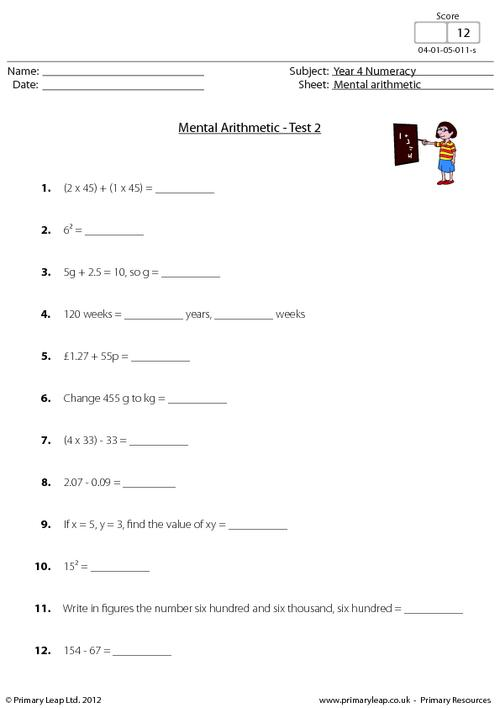### Mental arithmetic - Test 1

A year 4 numeracy worksheet with 12 mental arithmetic questions.

Age: 8 - 9
1
2
3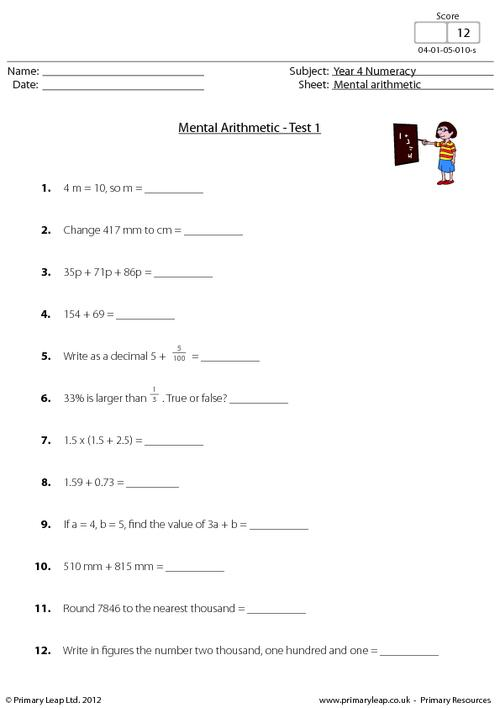### Division: 4 digits by 1 - set 7

Year 4 Maths: Division - This worksheet asks children to divide four-digit numbers by 9. (21 questions)

Age: 8 - 9
1
2
3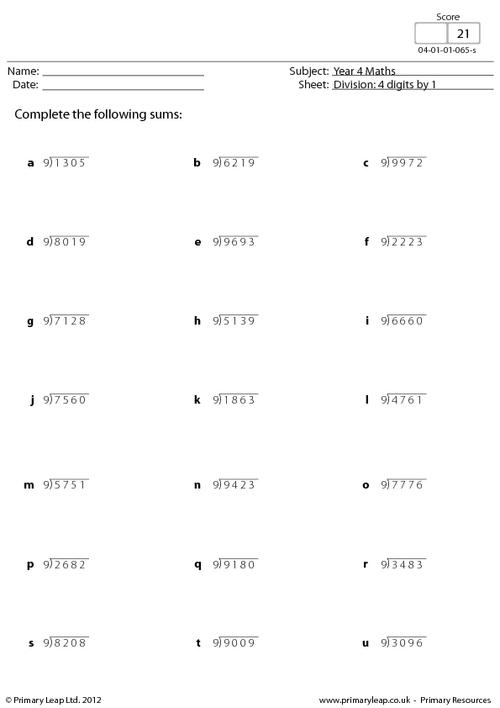of 7Our fun and engaging year 4 maths activities will help your child develop their mental and written calculation skills using larger positive numbers and fractions. Our maths worksheets will help your child learn to count in steps of 6, 7, 9, 25 and 1000, add and subtract four-digit numbers, multiply three-digit by one-digit numbers, and be able to divide by 10 and 100 to give decimal answers. Don’t worry if some of the methods are new to you, all of our maths worksheets come complete with answer sheets!Learn

One of the best things about printable worksheets is that they make learning fun and easy. Explore our educational resources based on Year 4 Maths and keep your children learning through these fun and engaging exercises.Progress

Our Year 4 Maths printable worksheets are designed for progressive learning. Our engaging content enables a child to learn at their own speed and gain confidence quickly. When we help a child believe in themselves, we empower them for life.Improve

Printable worksheets are a great way to enhance creativity and improve a child’s knowledge. Help your child learn more about Year 4 Maths with this great selection of worksheets designed to help children succeed.